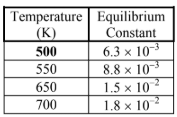# Problem: The table below lists the equilibrium concentrations for H 2(g), I2(g), and HI(g) in the given reaction.H2 (g) + I2 (g) ⇌ 2HI (g)The equilibrium partial pressures observed when the reaction comes to equilibrium at 720 K are PI2 = 0.467, PH2 = 0.467, and PHI = 0.666. What is the equilibrium constant for the reaction at this temperature? Please circle your answer.a. 0.327b. 0.701c. 2.03d. 1.42e. 1.88

###### FREE Expert Solution

We’re being asked to calculate the equilibrium constant for the reaction:

H2(g) + I2(g) ⇌ 2 HI(g);

We're given the equilibrium pressures:

PH2 = 0.467 atm
PI2 = 0.467 atm
PHI =  0.666 atm

The Kp expression for the reaction is:

90% (488 ratings)###### Problem Details

The table below lists the equilibrium concentrations for H 2(g), I2(g), and HI(g) in the given reaction.

H(g) + I(g) ⇌ 2HI (g)The equilibrium partial pressures observed when the reaction comes to equilibrium at 720 K are PI2 = 0.467, PH2 = 0.467, and PHI = 0.666. What is the equilibrium constant for the reaction at this temperature? Please circle your answer.

a. 0.327

b. 0.701

c. 2.03

d. 1.42

e. 1.88